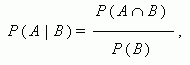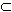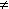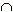Conditional probability. Independence of events

Conditional probability. Independent and dependent events.

The probability of occurrence of an event A at the condition, that an event B takes place, is called the conditional probability and calculated by the formula:where À , ÂÅ , Ð ( Â )0.

The events À , ÂÅ are independent events , if Ð ( ÀÂ ) = Ð ( À ) · Ð ( Â ).

Otherwise, the events À and Â are called dependent events .